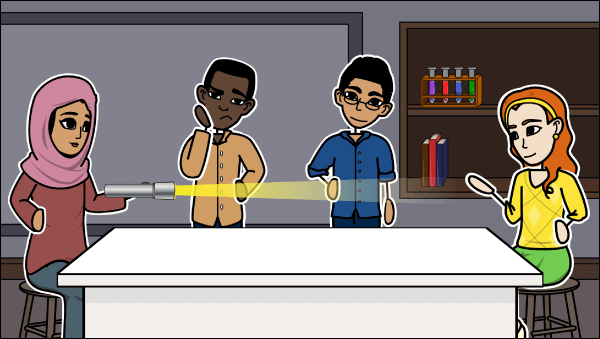Energy cannot be created or destroyed, and this concept is known as the conservation of energy. Because of this, the universe has a finite amount of energy which is transferred from one form to another. In the early 20th century, Einstein put forth the idea that energy and mass were interchangeable, leading to the most famous equation in Science: E=mc2. The activities in this lesson plan explore different forms of energy and energy transfers through the creation of visual aids.

## Teacher Background on Energy

The English physicist James Prescott Joule carried out several experiments that examined the equivalence of heat and mechanical energy (sum of potential and kinetic energy). He found that the temperature of water could be increased using mechanical energy. This led to the discovery of the law of conservation of energy, which states that the total energy in a closed system is constant, meaning energy can’t be created or destroyed.

For example, a light bulb transfers electrical energy to light energy. Light bulbs also get very warm, so not all the electrical energy is converted to light energy. Some of it is transferred into heat energy. We call this heat energy wasted energy and the light energy useful energy. Modern day light bulbs are more efficient than light bulbs were 50 years ago. This means that even with the same amount of electrical energy, more is transferred into light energy and less into heat energy. Engineers are working hard to increase the efficiency of many of the objects in our homes, so we use less electrical energy. Part of this effort is to help reduce the strain on energy resources. However, we need to look for new energy resources, as old methods of burning fossil fuels increases the greenhouse effect and has led to global warming.

### Types of Energy

#### Kinetic Energy

Kinetic Energy is also known as movement energy. This form of energy can be found in anything that moves, such as a car on a highway or a grasshopper jumping. The equation for kinetic energy is KE=½mv2. This signifies that the amount of kinetic energy depends on two factors: velocity and mass. If we increase both of these, then the kinetic energy will increase.

#### Sound Energy

Sound Energy is found in anything that vibrates. If the vibrations are between 20Hz and 20,000Hz, then they are said to be in the audible range and humans can hear them. Louder sounds (sound waves with bigger amplitudes) have more energy.

#### Thermal Energy

Thermal Energy is also known as heat energy. A hot cup of coffee has thermal energy. Over time, this thermal energy is dissipated to the surroundings as the coffee cools down. The amount of thermal energy is related to the temperature of an object.

#### Chemical Energy

Chemical Energy is energy that is stored in the chemical bonds between molecules and atoms. This energy can be released during a chemical reaction as sound, heat, light, or kinetic energy. An example of something that has chemical energy is food or a battery.

#### Electrical Energy

Electrical Energy can be found in moving or static charges. Electrical energy can be transferred into many different types of energy. With a television, electrical energy is transferred to light, sound, and heat energy.

#### Gravitational Potential Energy

Gravitational Potential Energy is stored energy in anything that has a height above the ground. A ball at the top of a tower has gravitational potential energy. As it falls, the gravitational potential energy is transferred to kinetic energy. The amount of gravitational potential energy depends on the mass of the an object, its height, and the strength of the gravitational field.

#### Light Energy

Light Energy is also known as radiant energy. It is found in all parts of the electromagnetic spectrum.

#### Elastic Potential Energy

Elastic Potential Energy is stored in things that are squashed or stretched, such as springs and rubber bands. The amount of energy stored depends on how compressed or stretched the object is, and how stiff the material is that the object is made of.

#### Nuclear Energy

Nuclear Energy is stored in the nuclei of atoms. It is released during nuclear reactions such as fusion and fission. Examples of this can be found in nuclear reactors and atomic bombs.

#### Magnetic Energy

Magnetic Energy is energy related to magnets or electromagnets. Maglev trains use magnetic energy to raise trains off the ground.